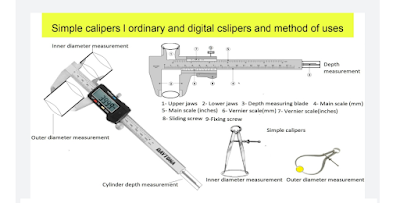## Tuesday, September 5, 2023

### Gears rotational speed and cylinder surface speed calculations ( spur gear, bevel gears, Worm, and worm gears)

Gears rotational speed calculation( spur gear, bevel gears, Worm, and worm gears)-step 1

Motor rpm(M) = 1400, Motor pulley dia(A) = 40 cms

Machine pulley dia(B)= 20 cms, Machine pulley rpm=? rpm

Rpm (B) = (Motor rpm(M) * Motor pulley dia(A)/Machine

pulley

dia(B)

=(1400 *40)/20

Machine pulley(B) rpm= 2800

Gears rotational speed calculation( spur gear,

bevel gears, Worm, and worm gears)-step 2

RPM gear( C ) = RPM ( B)= 2800, No. of teeth (gear C )

=25

No. of teeth (D )=60, RPM gear D =? RPM

RPM gear D = (RPM gear C * No. of teeth gear C )/No. of

teeth ( gear D )

=(2800 * 25)/60

RPM gear D = 1166.66

Gears rotational speed calculation( spur gear,

bevel gears, Worm, and worm gears)-step 3

RPM bevel gear E=RPM gear D=1166.66,

Since bevel gear E is mounted on gear shaft of D, therefore rpm

of bevel gear E = RPM of D = 1166.66

No, of teeth in bevel gear E and F are equal to each other,

therefore rpm of bevel gear F = RPM of bevel gear E = 1166.66

Gear G is mounted on F, therefore rpm of G = rpm of F=

1166.66

Gears rotational speed calculation( spur gear,

bevel gears, Worm, and worm gears)-step 4

Rpm gear G = 1166.66, No. of teeth grar G=25

No. of teeth gear H = 60, RPM of gear H = ?

RPM of gear H = (Rpm gear G * No. of teeth grar

G)/No. of teeth gear H

=(1166.66 *25)/60

RPM of gear H = 486.11

Gears rotational speed calculation( spur gear,

bevel gears, Worm, and worm gears)-step 5

Since the single worm I is mounted on the gear shaft of H,

therefore rpm of single worm I = rpm of gear H = 486.11

No of teeth in worm gear J = 42, rpm of worm gear J = ?

rpm of worm gear J = (rpm of single worm I * 1 ) / No of teeth

in worm gear J

rpm of worm gear J =(486.11* 1 ) / 42

rpm of worm gear J = 11.57

Gears rotational speed calculation( spur gear,

bevel gears, Worm, and worm gears)-step 6

Since the worm gear J and roller K are mounted upon same shaft,

therefore the rpm of Roller K = rpm of worm gear J = 11.57

Dia of roller K = 75 cms

Surface speed (K) = Rpm of K * 3.14 * dia of K

= 11.57 * 3.14 * 75

= 2724.73 CMS / Minute

Surface speed (K) = 27.25 meters / minte